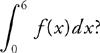# AP Calculus BC Practice Test 39

### Test Information8 questions24 minutes

Calculator Allowed

1. What is the acceleration vector of a particle at t = 2 if the particle is moving in the xy-plane and its velocity vector is v (t) = < t, 4t3> ?

2. The equation of the normal line to the graph y = e2x at the point where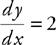is

3.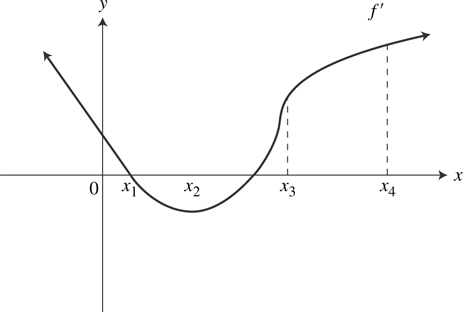The graph of f′, the derivative of f, is shown above. At which value of x does the graph of f have a point of inflection?

4. The temperature of a metal is dropping at the rate of g (t) = 10e-0.1t for 0 ≤ t ≤ 10, where g is measured in degrees in Fahrenheit and t in minutes. If the metal is initally 100°F, what is the temperature to the nearest degree Fahrenheit after 6 minutes?

5. A particle moves along the y-axis so that its position at time t is y (t) = 5t3 - 9t2 + 2t - 1. At the moment when the particle first changes direction, the (x, y) coordinates of its position are

6. The interval of convergence of the series is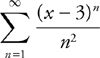is

7.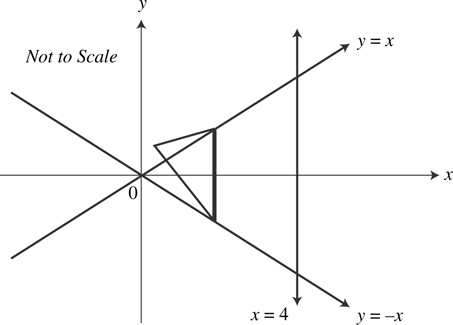The base of a solid is a region bounded by the lines y = x, y = -x, and x = 4, as shown above. What is the volume of the solid if the cross sections perpendicular to the x-axis are equilateral triangles?

8. Let f be a continuous function on [0, 6] and have selected values as shown below.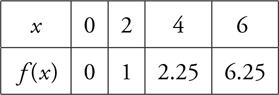If you use the subintervals [0, 2], [2, 4], and [4, 6], what is the trapezoidal approximation of